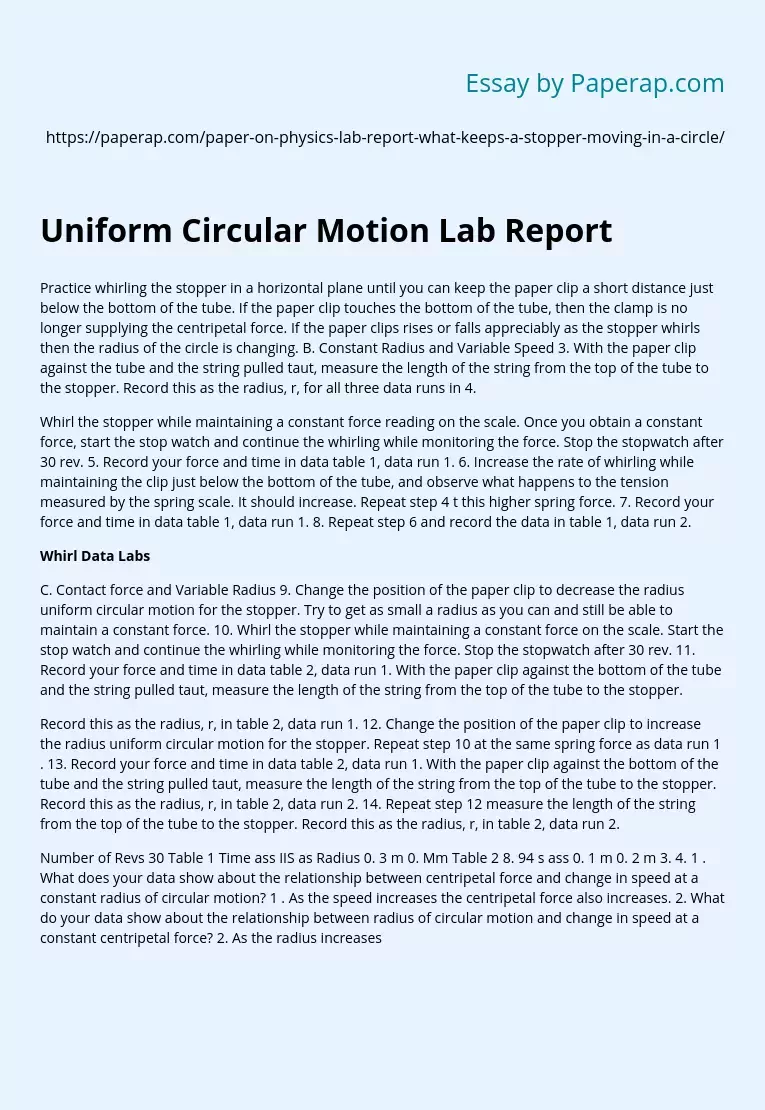# Uniform Circular Motion Lab Report

Practice whirling the stopper in a horizontal plane until you can keep the paper clip a short distance just below the bottom of the tube. If the paper clip touches the bottom of the tube, then the clamp is no longer supplying the centripetal force. If the paper clips rises or falls appreciably as the stopper whirls then the radius of the circle is changing. B. Constant Radius and Variable Speed 3. With the paper clip against the tube and the string pulled taut, measure the length of the string from the top of the tube to the stopper.

Record this as the radius, r, for all three data runs in 4.

Whirl the stopper while maintaining a constant force reading on the scale. Once you obtain a constant force, start the stop watch and continue the whirling while monitoring the force. Stop the stopwatch after 30 rev. 5. Record your force and time in data table 1, data run 1. 6. Increase the rate of whirling while maintaining the clip just below the bottom of the tube, and observe what happens to the tension measured by the spring scale.

It should increase. Repeat step 4 t this higher spring force. 7. Record your force and time in data table 1, data run 1. 8. Repeat step 6 and record the data in table 1, data run 2.

## Whirl Data Labs

C. Contact force and Variable Radius 9. Change the position of the paper clip to decrease the radius uniform circular motion for the stopper. Try to get as small a radius as you can and still be able to maintain a constant force.

Get quality help nowwriter-marianVerified

Proficient in: Force4.8 (309)

“ Writer-marian did a very good job with my paper, she got straight to the point, she made it clear and organized ”+84 relevant experts are online

10. Whirl the stopper while maintaining a constant force on the scale. Start the stop watch and continue the whirling while monitoring the force. Stop the stopwatch after 30 rev. 11. Record your force and time in data table 2, data run 1. With the paper clip against the bottom of the tube and the string pulled taut, measure the length of the string from the top of the tube to the stopper.

Record this as the radius, r, in table 2, data run 1. 12. Change the position of the paper clip to increase the radius uniform circular motion for the stopper. Repeat step 10 at the same spring force as data run 1 . 13. Record your force and time in data table 2, data run 1. With the paper clip against the bottom of the tube and the string pulled taut, measure the length of the string from the top of the tube to the stopper. Record this as the radius, r, in table 2, data run 2. 14. Repeat step 12 measure the length of the string from the top of the tube to the stopper. Record this as the radius, r, in table 2, data run 2.

Number of Revs 30 Table 1 Time ass IIS as Radius 0. 3 m 0. Mm Table 2 8. 94 s ass 0. 1 m 0. 2 m 3. 4. 1 . What does your data show about the relationship between centripetal force and change in speed at a constant radius of circular motion? 1 . As the speed increases the centripetal force also increases. 2. What do your data show about the relationship between radius of circular motion and change in speed at a constant centripetal force? 2. As the radius increases so does the speed of the stopper. 3. Analysis the resulting plot. Is there a pattern? If so what does the pattern mean? 4.

Formulate an equation for the relationship between the spring force and the centripetal acceleration of the stopper. What does this equation tell you about the spring force? This shows that the spring force is in direct relation to the centripetal force. 5. Justify the method of recording time for 30 rev to minimize the error associated with calculating the speed of the stopper. In other labs you have used multiple data runs and averaged the data to minimize the random error associated with measuring. Why did you not have to do that on 5. To get the most accurate time we had the person timing also count the revolutions of the stopper.

And we didn’t have to do multiple data runs because the time is the least important In this lab we found that the variables of circular motion are directly related. We accomplished our goal which was to relate the variables involved in uniform circular motion. I also proved my hypothesis incorrect, my hypothesis was that the velocity of the stopper will increase as the radius is shortened, which in fact as the radius was shortened the velocity decreased. In this lab there were several sources of error, the stopper was spinning so fast we may have miss counted how many revolutions it had, and the force was not constant the entire time.